Courses
Courses for Kids
Free study material
Offline Centres
More

# Chemical Bonding and Molecular Structure Class 11 Notes CBSE Chemistry Chapter 4 (Free PDF Download)Last updated date: 04th Dec 2023
Total views: 676.8k
Views today: 13.76k

## Revision Notes for CBSE Class 11 Chemistry Chapter 4 (Chemical Bonding and Molecular Structure) - Free PDF Download

Students generally think Class 11th Chemistry is tough and good marks cannot be scored in this subject, but this doesn't seem right. With proper guidance and with appropriate notes, good marks can easily be achieved in Chemistry in Class 11th. There are many important, and easy chapters are there in Class 11 Chemistry which can easily help students attain good marks in exams. One such chapter is Chapter 4 - Chemical Bonding and Molecular Structures. Revision notes of Chapter 4 Chemistry Class 11 can help students to get good marks in Class 11th examinations as they cover all the necessary topics, concepts and terms in a fun and simple language for the proper understanding of students.

During the examination Class 11th Chemistry Chapter 4 notes about Chemical Bonding and Molecular Structures become very important. These notes are prepared by some expert Chemistry teachers. Through chemical bonding Class 11 notes, it becomes easy for the students to know all the essential things that are present in the chapter. Revision notes become a crucial thing for those students who are actually serious about scoring good grades.

Also, check CBSE Class 11 Chemistry revision notes for other chapters:

## Chemical Bonding and Molecular Structure Chapter Related Important Study MaterialsIt is a curated compilation of relevant online resources that complement and expand upon the content covered in a specific chapter. Explore these links to access additional readings, explanatory videos, practice exercises, and other valuable materials that enhance your understanding of the chapter's subject matter.

 Chemical Bonding and Molecular Structure Related Other Study Materials Chemical Bonding and Molecular Structure Important Questions Chemical Bonding and Molecular Structure NCERT Solutions Chemical Bonding and Molecular Structure NCERT Exemplar

## Chemical Bonding and Molecular Structure Class 11 Notes Chemistry - Basic Subjective Questions

### Section – A (1 Mark Questions)

1. The given statement is True or False:

The shape of SF6 molecule is octahedral whereas that of IF7 is square pyramidal.

Ans. False shape of IF7 is pentagonal bipyramidal

2. Why is NaCl a bad conductor of electricity in the solid state?

Ans. In the solid state, Na+ and Cl ions are strongly held together and are not free to move.

3. Out of HF, HCl, HBr and HI, the lowest boiling point is of ________ and highest boiling point is of ________.

Ans. Out of HF, HCl, HBr and HI, the lowest boiling point is of HCl and highest boiling point is of HF.

4. The bond in peroxide ion is _________ then in superoxide ion.(Weaker /Stronger)

Ans. Weaker

5. In N2 molecule energy of π2px and π2py are ________ then σ2pz. (Lower/Higher)

Ans. Lower

6. N2 molecule is __________. (Paramagnetic/Diamagnetic)

Ans. Diamagnetic

7. Give one example each for a compound with

(a) An ionic bond

(b) A covalent bond

Ans. (a) Sodium chloride

(b) Methane (CH4)

8. What is the SI unit of dipole moment?

Ans. Coulomb meter (Cm).

9. Give two examples of molecules containing coordinate bonding.

Ans. H3O+, O3

10. Name one compound each involving sp3, sp2, sp hybridization.

Ans. sp3: CH4 ; sp2: C2H4 ; sp: C2H2

### Section – B (2 Marks Questions)

11. Write the significance of plus and minus sign shown in representing the orbitals.

Ans. Molecular orbitals are represented by wave functions. A plus sign in an orbital indicates a positive wave function while a minus sign in an orbital represents a negative wave function.

12. Define electronegativity. How does it differ from electron gain enthalpy?

Ans. Electronegativity: Electronegativity is the tendency of an atom to attract shared pair of electrons towards itself. It is the property of bonded atom. Whereas electron gain enthalpy is the tendency of an atom to attract outside electron. It is the property of an isolated atom.

13. A molecule of PCl5 exists while that of NCl5 does not.

Ans. PCl5 forms five bonds by using the d-orbitals to “expand the octet” and have more places to put bonding pairs of electrons. NCl5 does not exist because there are no d-orbitals. Therefore, there is no way to arrange five pairs of bonding electrons around a nitrogen atom.

14. What is difference between bond order and bond length and state relation between them?

Ans. Bond order is the number of bonds between two atoms while bond length is the equilibrium distance between the nuclei of two bonded atoms in a molecule. Higher the bond order smaller will be the bond length.

15. Identify the compound/compounds which does not obey octet rule:
LiCl, SF6, H2SO4.

Ans. Due to incomplete octet, LiCl does not obey octet rule. SF6, H2SO4 as both have more than eight valence electrons around the central atom.  So, the octet rule does not apply in such cases.

16. Which of the following has maximum bond angle?
H2O, CO2, NH3, CH4

Ans. CO2, due to its linear structure. CO2, is sp hybridised and have bond angle equal to 180º

17. List two conditions for forming hydrogen bonds.

Ans. (i) High electronegativity of atom bonded to hydrogen

(ii) Small size of the electronegative atom.

18. Write down the M.O. configuration of N2 and calculate its Bond order.

Ans. Nitrogen molecule(N2) – The total number of electrons = 14 and filling in molecular orbitals we have

$\sigma1\text{s}^2<\sigma^*1\text{s}^2<\sigma2\text{s}^2<\sigma^*2\text{s}^2<\pi\text{2p}^2_x=\pi\text{2p}^2_y<\sigma\text{2p}^2_z$

$\text{Bond order}=\frac{\text{N}_\text{b}-\text{N}_\text{a}}{2}=\frac{10-4}{2}=\frac{6}{2}=3$

It is diamagnetic.

19. Why does type of overlap given in the following figure not result in bond formation ?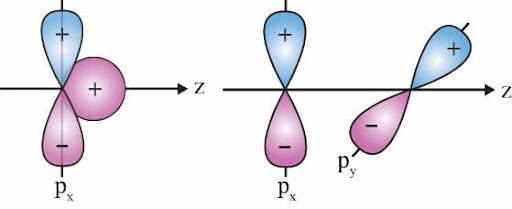Ans. When the orientation of two interacting atomic orbital is such that there is no overlapping of the orbital, that is known as zero overlapping. In the given two diagrams, the interacting orbitals do not overlap with each other ,so there is no overlapping and no bond formation.

20. AlF3 is a high melting solid whereas SiF4 is a gas. Explain why?

Ans. AlF3 is an ionic solid due to the large difference in electronegativities of Al and F whereas SiF4 is a covalent compound and hence there are only weak van der Waal’s forces among its molecules.

PDF Summary - Class 11 Chemistry Chemical Bonding and Molecular Structure Notes (Chapter 4)

1. Introduction:

Atoms are usually not capable of free existence but groups of atoms of the same or different elements exist as one species, e.g., $\mathrm{H}_{2} \mathrm{O}_{2}, \mathrm{P}_{4} \mathrm{~S}_{\mathrm{s}}, \mathrm{H}_{2} \mathrm{O}$. A molecule is a collection of atoms that exist as a single species and share common features. Clearly, some force is at work within the molecules to keep these atoms together.

### 2. Chemical Bond:

Chemical bonding is the creation of a chemical compound by forming a chemical link between two or more atoms, molecules, or ions. The atoms in the resulting molecule are held together by chemical bonds.

#### Types of Chemical Bonds Include:

1. Ionic Bonds

2. Covalent Bonds

3. Hydrogen Bonds

4. Polar Bonds

#### Modes of Chemical Composition:

1. When one or more electrons are completely transferred from one atom to another. Electrovalency is the name given to this process, and the chemical bond generated is known as an electrovalent bond or an ionic bond.

2. Through the sharing of electrons. This can happen in one of two ways:

1. A covalent bond is created when the two joining atoms provide equal amounts of shared electrons.

2. A coordinate bond, also known as a dative bond, is produced when these electrons are contributed totally by one of the atoms but shared by both.

### 3. Lewis Symbols:

In 1916, Kossel and Lewis were successful in providing a successful explanation for why atoms unite to form molecules based on the concept of an electrical configuration of noble gases. Noble gas atoms have little or no inclination to mix with other noble gas atoms or atoms of other elements. This implies that the electronic arrangements of these atoms must be stable.

Lewis used simple symbols to represent an atom's valence shell electrons. The outer shell electrons are depicted as dots around the atom symbol. Lewis symbols or electron dot symbols are the names given to these symbols.

4. Ionic Bond:

Ionic bonding is a sort of chemical bonding that occurs when electrons are transferred from one atom or molecule to another. One atom loses an electron in this process, which is then gained by another atom. When an electron transfer occurs, one of the atoms gains a negative charge and is referred to as an anion.

The cation is the other atom that gets a positive charge. The difference in charge between the two atoms strengthens the ionic link; the higher the charge differential between the cation and the anion, the stronger the ionic bond.

Explanation of Ionic Bond: Atoms are electrically neutral. Therefore, they possess an equal number of protons and electrons. On losing an electron, an atom becomes positively

$\mathrm{A} \longrightarrow \mathrm{A}^{+}+\mathrm{e}^{-}$

On the other hand, in case of atoms gaining the electron, the number of electrons exceeds the number of protons and thus the atom becomes negatively charged.

$\mathrm{B}+\mathrm{e}^{-} \longrightarrow \mathrm{B}^{-}$

The oppositely charged particles formed above attract each other by electrostatic forces of attraction. The bond thus formed is known as electrovalent or ionic bond.

#### Electrovalency:

The electrovalency of an element is defined as the number of electrons lost or gained during the development of an electrovalent connection.

For example, sodium and calcium both lost one electron and had valencies of one and two, respectively. Chlorine and oxygen gain one and two electrons, respectively, and have an electrovalency of one and two. In other words, valency is the same as the ion's charge.

#### Factors Governing the Formation of Ionic Bonds:

1. Ionisation Enthalpy (Ionization Energy): The amount of energy necessary to remove one electron from the outermost shell of an isolated atom in gaseous phase and convert it to a gaseous positive ion is known as ionisation enthalpy.

2. Electron Gain Enthalpy (Electron Affinity): The enthalpy change that occurs when an extra electron is added to an isolated atom in the gaseous phase to form a gaseous negative ion is known as electron affinity.

3. Lattice Enthalpy (Lattice Energy): In the production of ionic compounds, positively and negatively charged ions combine to form the compound.

#### Characteristics of Ionic Compounds:

1. Crystalline in Nature: Ionic compounds form crystalline structures, but the size of the crystalline structure is determined by the size of the anions and cations. Because the number of cations equals the number of anions that combine to form ionic compounds, the crystal structure of ionic compounds is uncharged.

2. Ionic compounds are stiff and brittle because the ions are bound together by strong electrostatic forces. Because these compounds are held together by strong forces, breaking them requires a lot of force. Ionic compounds are fragile by nature.

3. Boiling and melting points are also high.

Ionic compounds are hard to break because they are kept together by strong electrostatic forces. High melting and boiling temperatures are required to break such strong forces and overcome the force of attraction between them.

1. High Fusion and Vaporization Enthalpies: Ionic chemicals have higher Fusion and Vaporization Enthalpies than other molecular molecules. Their enthalpies are ten to one hundred times higher than those of molecular molecules.

2. Solubility: Ionic compounds can be dissolved in polar solvents such as water. In Non-Polar Covalent solvents, they are less soluble.

3. Good Conductors of Electricity in Aqueous or Molten Form: Ionic compounds act as a good conductor of electricity when they are in aqueous or molten form because ionic compounds are tightly packed, which prevents ions from moving, making them a poor conductor of electricity. However, when they are in aqueous or molten form, ions are free to move, making them a good conductor of electricity.

4. Acts as Insulators: When molten or watery, ionic compounds act as good conductors of electricity; otherwise, they act as insulators.

### 5. Covalent Bond:

In nature, covalent bonds can be either polar or nonpolar. Electrons are shared unequally in Polar Covalent chemical bonding because the more electronegative atom pulls the electron pair closer to itself and away from the less electronegative atom. A polar molecule like water is an example.

The irregular spacing of electrons between the atoms causes a charge difference in different parts of the atom. The molecule's one end is partially positively charged, while the other end is partially negatively charged.

Example: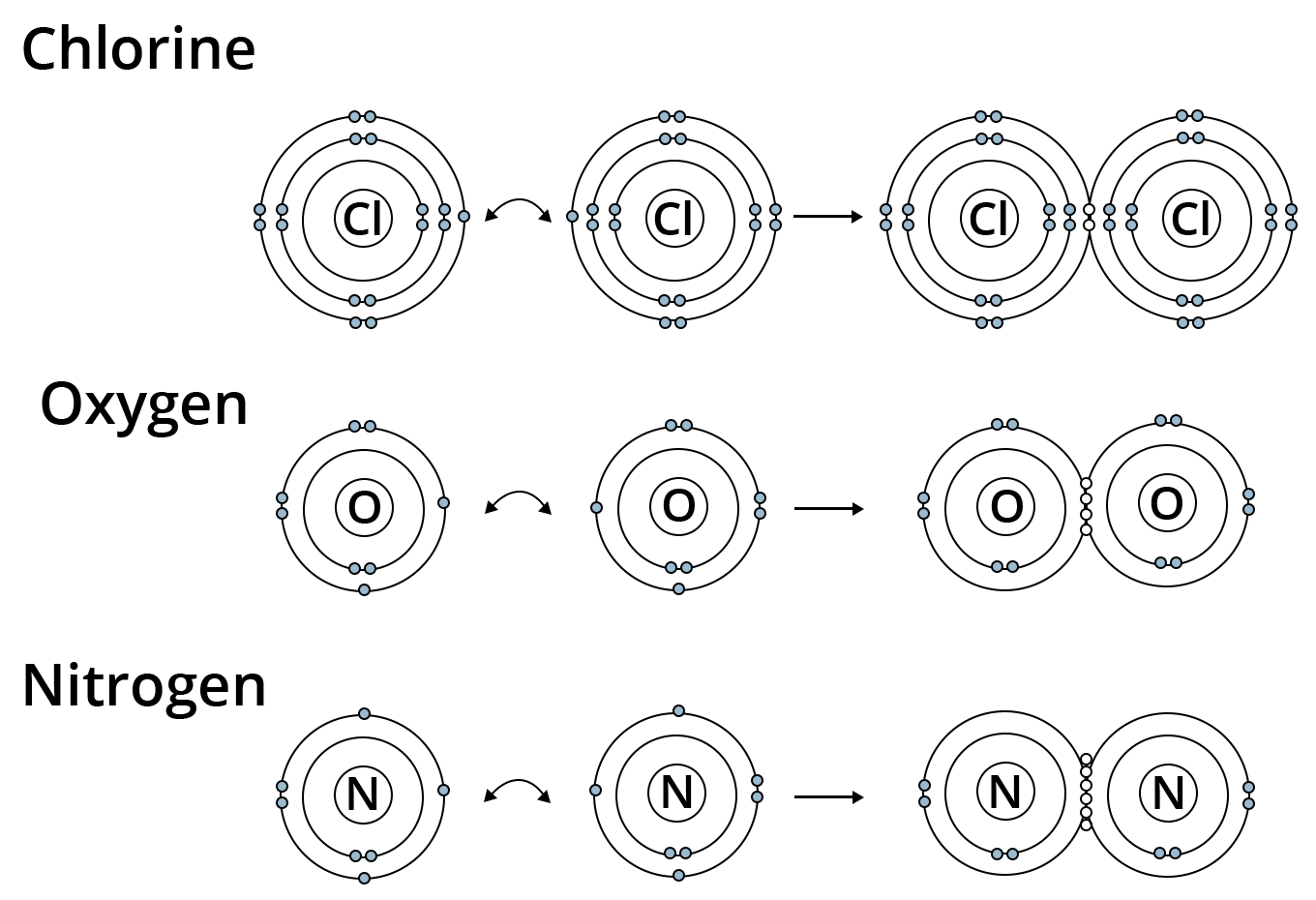Showing Covalent bonding in Chlorine, Oxygen and Nitrogen.

#### Conditions for Writing the Lewis Dot Structures:

• Sharing of an electron pair between the atoms results in the formation of covalent bonds.

• During bond formation, each bond consists of two electrons which are contributed by each one of the combining atoms.

• By the mutual sharing of electrons, each atom attains octet configuration in its valence shell.

The octet rule is used to write electron dot structures of covalent compounds. Except for the hydrogen atom, all of the atoms in the molecule will have eight electrons in their valence shell according to this law. Because only two electrons complete the first shell of hydrogen to achieve the helium configuration, it will have only two electrons.

To achieve a stable octet, elements in group 17 like Cl would share one electron; elements in group 16 like O and S would share two electrons; elements in group 15 would share three electrons, and so on.

For Example, the oxygen atom which has six electrons in its valence shell completes its octet by sharing its two electrons with two hydrogen atoms to form a water molecule.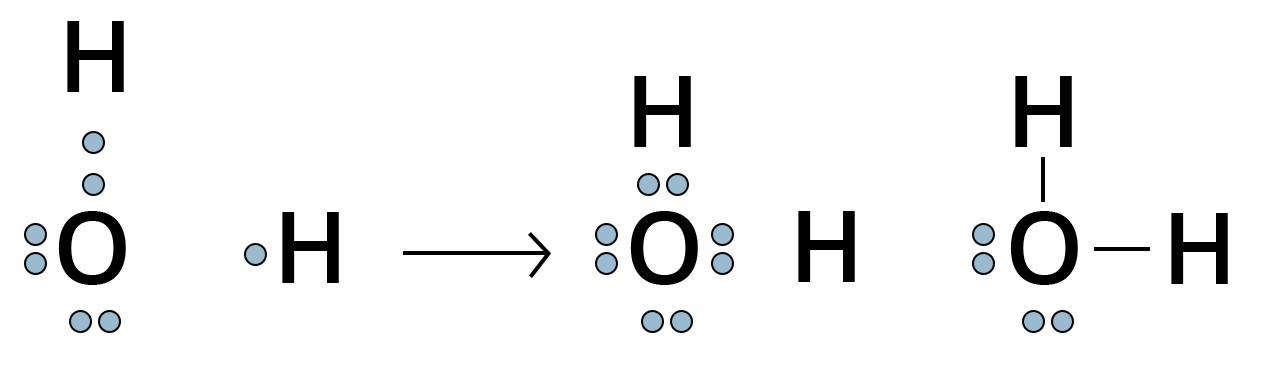Showing Lewis DOT Structure of Water Molecule

### 6. Formal Charge:

A charge on an individual atom(s) in a polyatomic molecule is known as formal charge. It's a deceptive charge that's linked to only one atom in the structure. We term it fake because a molecule or compounds true charge is distributed across the species' structure. Formal charge is caused by flaws in the configuration of an atom involved in the synthesis of a compound. Calculation is required for formal charge, as we will see later. From here, we'll go through things like the formal charge formula and how to compute formal charge, among other things. The formal charge is a crucial part in chemistry.

#### Formal Charge Formula:

Now that we know what formal charge is, we will move onto the formal charge formula. Mathematically, the formal charge formula stands as follows:

Formal Charge = Valence Electrons - 0.5 Bonding Electrons - Nonbonding Electrons

We half the value of bonding electrons because the bond exists between two electrons.

Now that we know the formal charge formula, we can move onto an example and understand how to calculate formal charge of a polyatomic molecule.

Example:

How To Calculate Formal Charge Of $\text{S}{{\text{O}}_{\text{2}}}$?

Here we will understand how to calculate the formal charge of $\text{S}{{\text{O}}_{\text{2}}}$. For calculating the formal charge of $\text{S}{{\text{O}}_{\text{2}}}$, we have to unlock its Lewis structure. Therefore, the first step of calculating formal charge is drawing the Lewis structure. The Lewis structure of $\text{S}{{\text{O}}_{\text{2}}}$ is as follows:

Numbers 1,2,3,4 Indicate The Index of the Oxygen Atom.

 Atom Valence Electrons in the Free State No. of Non-Bonding Electrons in Lewis Structure No. of Pairs of Bonding Electrons in Lewis Structure Formal Charge Sulphur (S) 6 0 12 6 – 0 – 12/2 = 0 Oxygen (O) – 1 6 4 4 6 – 4 – 4/2 = 0 Oxygen (O) – 2 6 6 2 6 – 6 – 2/2 = -1 Oxygen (O) – 3 6 4 4 6 – 4 – 4/2 = 0 Oxygen (O) - 4 6 6 2 6 – 6 – 2/2 = -1

The above example shows us how to calculate the formal charge of $\text{S}{{\text{O}}_{2}}$. Therefore, we need to know the Lewis structure of the molecule or ion, and then we apply the formula for calculating formal charge.

Write the Formal Charges on Atoms in Nitrite Ions.

Lewis structure of $\text{N}{{\text{O}}_{2}}-$ ion is;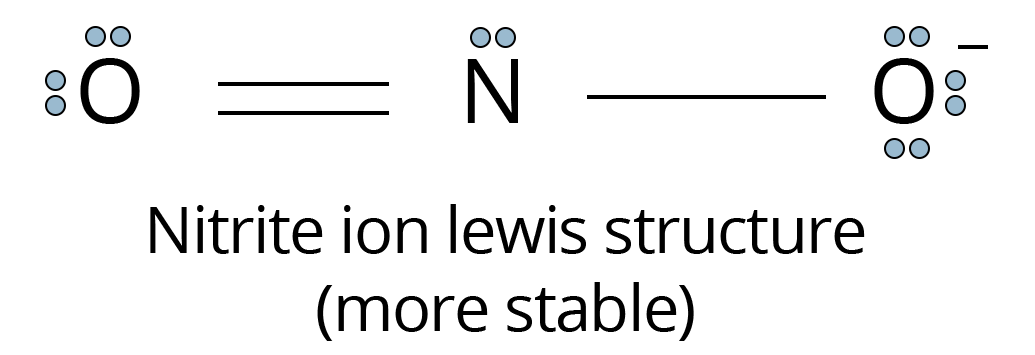Lewis structure of NO2 ion

Formal charge on N atom

=5 - ( - 1) = 6

Formal charge on double bonded O atom

= 6 - 4 - 0.5(4) = 0

Formal charge on single bonded O atom

= 6 - 6 - 0.5 (2) = -1

#### Significance of Formal Charge:

The fundamental benefit of calculating formal charges is that it aids in the selection of the most stable structure, i.e. the one with the least energy, among the several Lewis structures. The one with the least formal charges on the atoms is the most stable.

7. Limitations Of Lewis Langmuir Concept Of Covalent Bond:

Lewis-Langmuir concept of covalent bond, as explained in above, has the following limitation:

• It could not explain how the atoms are held together in the molecules like $\mathrm{H}_{2}, \mathrm{Cl}_{2}$ etc. in which there are no ions and hence there are no electrostatic forces of attraction, i.e., it could not explain the formation of a covalent bond.

• It could not explain the shapes of molecules containing covalent bonds.

• It could not explain the release of energy during the formation of a covalent bond.

8. Valence Shell Electron Pair Repulsion Theory:

VSEPR (pronounced "vesper") stands for valence shell electron pair repulsion model, which is a model for predicting the geometry of molecules. VSEPR models examine the bonding and molecular geometry of organic compounds and polyatomic ions in particular. It is applicable to practically all compounds with a non-metal centre atom.

Because Lewis structures are limited to two dimensions, they can only tell you the number and type of links between atoms. The VSEPR model accurately predicts the 3-D shape of molecules and ions, but it fails to provide any detailed information on link length or bond structure.

The following Terms are Commonly Used in Discussing the Shapes of Molecules:

• VSEPR models are based on the idea that electrons revolving around a core atom will arrange themselves to minimise repulsion, dictating the molecule's shape.

• As long as the central atom is not a metal, it can predict the form of practically all compounds with a central atom. Each form is given a name as well as an idealised bond angle.

• Lone pair: A pair of valence electrons that are not shared with another atom.

• Bond Angle: The angle formed by a bonded atom, the centre atom, and another bonded atom

• Molecular Geometry: A polyatomic ion or molecule's 3-D arrangement of bound atoms.

• Electron Pair Geometry is the 3-D arrangement of electron pairs around a polyatomic ion or molecule's core atom.

The major distinction between molecular geometry and electron pair geometry is that molecular geometry excludes unpaired electrons, whereas electron pair geometry includes both bound and unpaired atoms. The molecule and electron pair geometries will be the same if there are no unpaired electrons in the chemical under consideration.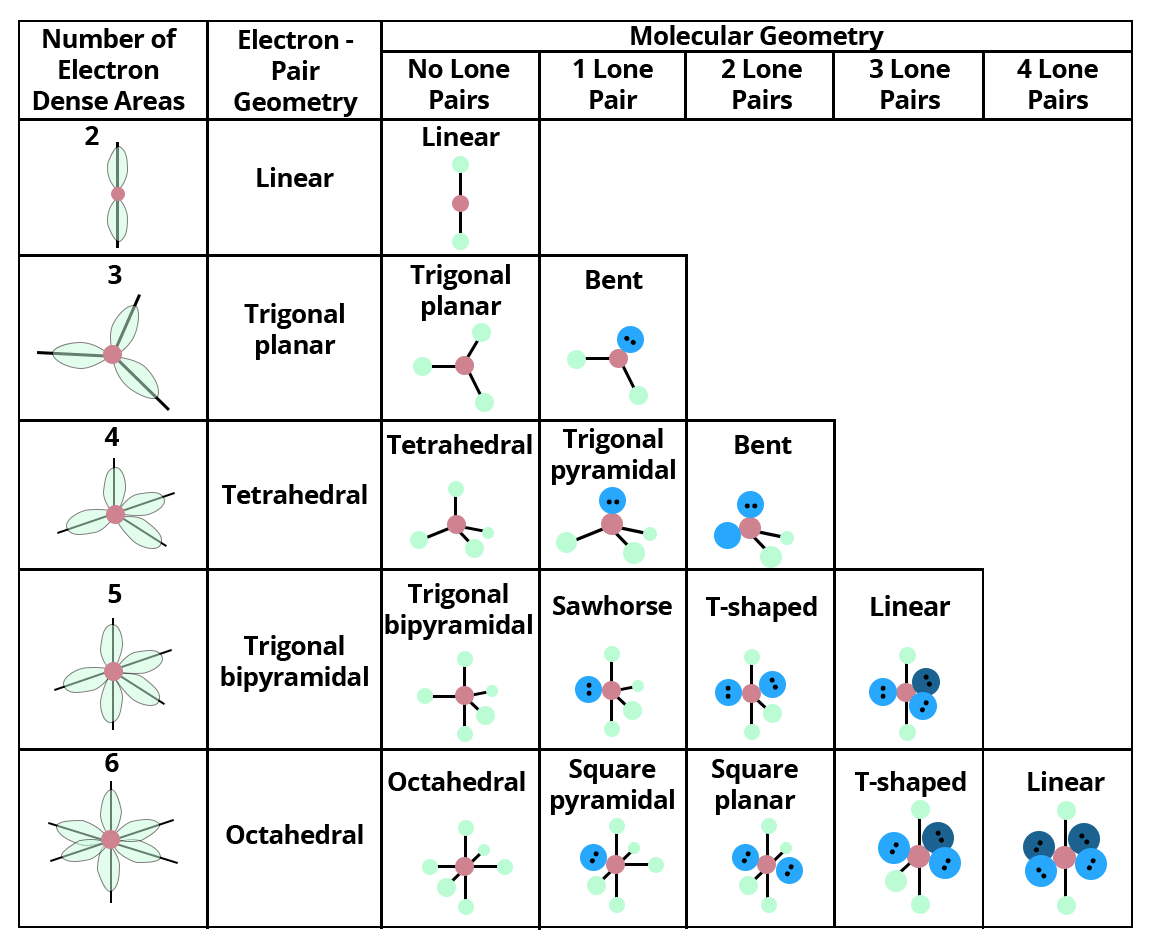Showing valence shell electron pair repulsion model for predicting the geometry of molecules

Calculating the total number of electron pairs, bond pairs, and lone pairs, as well as estimating molecule and ion shapes:

1. Total no. of electron pairs around the central atom =   1 (No. of valence electrons of central atom + No. of atoms linked to central atom by single   bonds)

For negative ions, add the number of electrons equal to the units of negative charge on the ion to the valence electrons of the central atom.

For positive ions, subtract the number of electrons equal to the units of positive charge on the ion from the valence electrons of the central atom.

1. No. of bond pairs (shared pairs) = No. of atoms linked to the central atom by single bonds.

2. No. of lone pairs = Total no. of electron pairs – No. of shared pairs.

On the Basis of VSEPR Theory, predict the Shapes of the Following:

(i) $\mathrm{ClF}_{3}$

(ii) $\mathrm{BrF}_{5}$

Sol. (i) Shape of ClF $_{3}$

No. of valence electrons of the central $\mathrm{Cl}$ atom $=7$

No. of atoms linked to it by single bonds $=3$.

$\therefore \quad$ Total no. of electron pairs around $\mathrm{Cl}=\frac{7+3}{\mathrm{Cl}}=5$

No. of bond pairs $=$ No. of atoms linked to $\mathrm{Cl}=3$.

$\therefore \quad$ No. of lone pairs $=5-3=2$

Thus, the molecule is of the type $\mathrm{AB}_{3} \mathrm{~L}_{2}$.

Hence, it is T-shaped.

(ii) Shape of $\mathrm{BrF}_{5}$

No. of valence electrons of central Br atom $=7$

No. of atoms linked to it by single bonds $=5$.

$\therefore \quad$ Total no. of electron pairs around $\mathrm{Br}=\frac{7+5}{}=6$

No. of bond pairs $=$ No. of atoms linked to $\mathrm{Br}=5$.

$\therefore \quad$ No. of lone pairs $=6-5=1$.

Thus, the molecule is of the type $\mathrm{AB}_{5} \mathrm{~L}$. Hence, it has a square pyramidal shape.

### 9. Hybridisation:

Hybridisation is defined as the mixing of atomic orbitals belonging to the same atom but with slightly varying energies, resulting in an energy redistribution between them and the production of new orbitals with identical energies and shapes. Hybrid orbitals are the novel orbitals that result from this process.

Hybridisation: Some Important Points:

1. Only orbitals with comparable energies and belonging to the same atom or ion can be hybridised.

2. The number of hybrid orbitals created equals the total number of atomic orbitals combined.

3. Hybridization does not require the participation of all half-filled orbitals. Similarly, only half-filled orbitals are not required to participate in hybridisation. Even orbitals that are totally filled but have slightly varying energy can participate.

4. Hybridisation does not happen in isolated atoms; it only happens when bonds are formed.

5. The type of hybridisation indicates the molecule's geometry. The type of hybridisation used can be used to determine the shape of a molecule.

6. The hybrid orbital is larger lobe is always positive, whereas the smaller lobe on the opposite side is always negative.

#### Type of Hybridisation:

1. Diagonal or sp Hybridisation: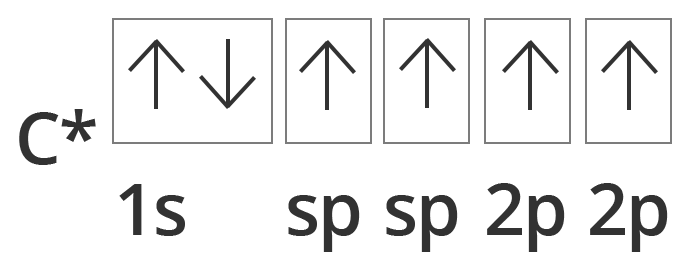Showing excited sate orbital filling in case of Carbon in case of  Diagonal or sp hybridisation

The chemical bonding in compounds such as alkynes with triple bonds is explained by sp hybridization. In this model, the 2s orbital is mixed with only one of the three p orbitals,

Resulting in two sp orbitals and two remaining p orbitals. The chemical bonding in acetylene (ethyne) $C_2H_2$ consists of sp–sp overlap between the two carbon atoms forming a σ bond and two additional π bonds formed by p–p overlap. Each carbon also bonds to hydrogen in a σ s–sp overlap at 180° angles.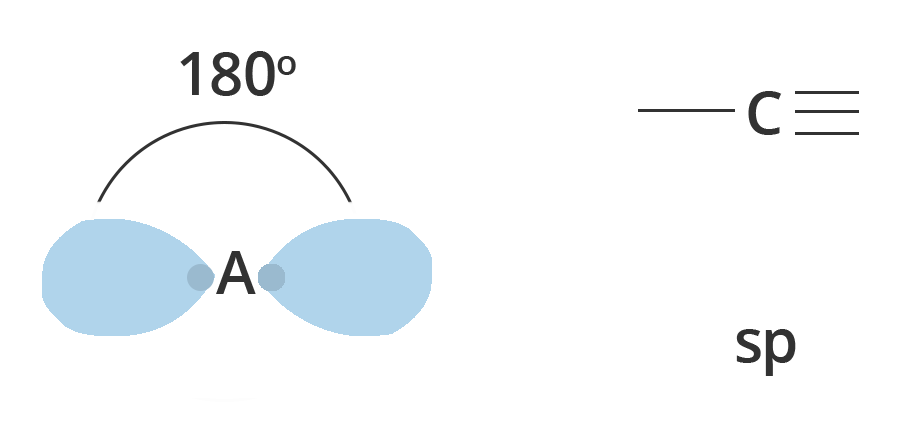Showing Diagonal or sp hybridisation

1. Diagonals of $\text{S}{{\text{p}}_{2}}$ Hybridization:

Other carbon compounds and other molecules may be explained in a similar way. For example, ethane ${{\text{C}}_{\text{2}}}{{\text{H}}_{\text{4}}}$ has a double bond between the carbons.

For this molecule, carbon $\text{S}{{\text{p}}_{2}}$ hybridises, because one π (pi) bond is required for the double bond between the carbons and only three σ bonds are formed per carbon atom. In $\text{S}{{\text{p}}_{2}}$ hybridisation the 2s orbital is mixed with only two of the three available 2p orbitals, usually denoted 2px and 2py. The third 2p orbital (2pz) remains unhybridized.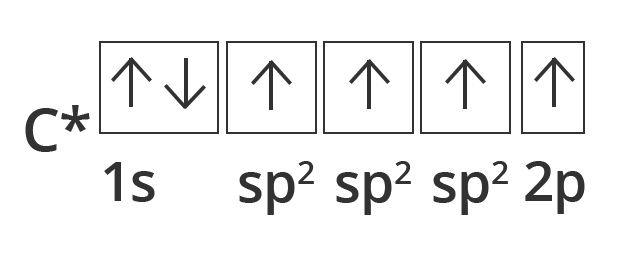Showing excited sate orbital filling in case of Carbon in case of Diagonal or Sp2 hybridisation.

Forming a total of three $\text{S}{{\text{p}}_{2}}$ orbitals with one remaining p orbital. In ethylene (ethane) the two carbon atoms form a σ bond by overlapping one $\text{S}{{\text{p}}_{2}}$ orbital from each carbon atom. The π bond between the carbon atoms perpendicular to the molecular plane is formed by 2p–2p overlap. Each carbon atom forms covalent C–H bonds with two hydrogens by s–$Sp_2$ overlap, all with 120° bond angles. The hydrogen–carbon bonds are all of equal strength and length, in agreement with experimental data.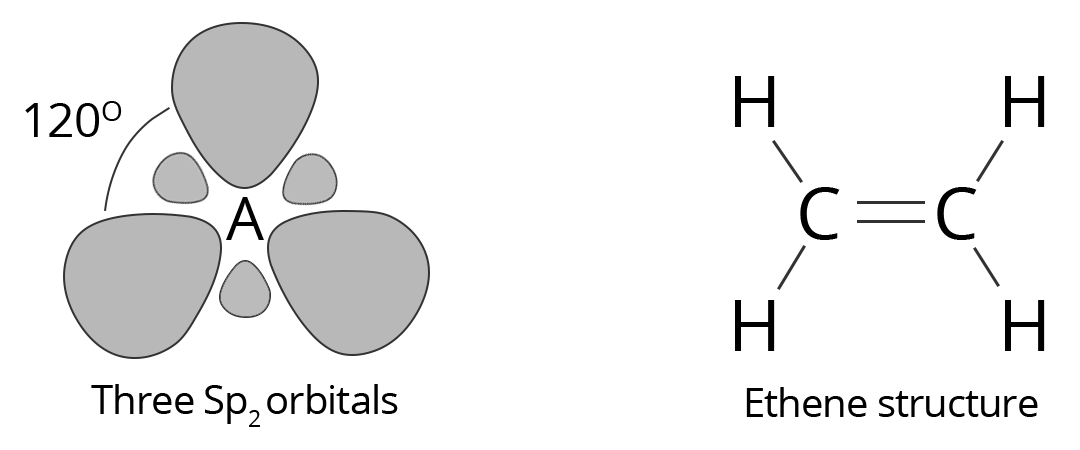Showing Diagonals of Sp2 hybridization

 Formula Shape(Name) Bond Angles Hybridization AX₂ Linear 180 sp AX₃ Trigonal Planar 120 sp² AX₄ Tetrahedral 109.5 sp³ AX₅ Triangular Bipyrimidal 120, 90 sp³d AX₆ Octahedral 90 sp³d² AX₂N Bent 120 sp² AX₂N₂ Bent 109.5 sp³ AX₃N Trigonal Pyramidal 109.5 sp³

### 10. Valence Bond Theory:

#### Types of Covalent Bond:

1. Sigma (σ) Bond.

2. Pi (π) Bond.

#### 1. Sigma (σ) Bond:

Head-on positive (same phase) overlap of atomic orbitals along the internuclear axis forms this sort of covalent connection. Due to the direct overlapping of the participating orbitals, sigma bonds are the strongest covalent bonds. The electrons that participate in a bond are referred to as electrons.

All single bonds are, in general, sigma bonds. The following atomic orbital combinations can be used to make them.

S-S Overlapping:

One “s” orbital from each participating atom undergoes head-on overlapping along the internuclear axis in this type of overlapping. Before one s orbital can overlap with another, it must be half-filled.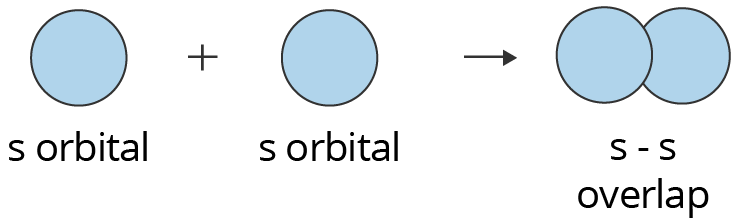Showing s-s Overlapping to form Sigma (σ) Bond

Above is an illustration of two s orbitals overlapping to form a sigma bond. In hydrogen molecules, where each hydrogen atom has a half-filled s orbital, this form of overlap happens.

Sp Overlapping:

Here, one half filled s orbital overlaps with one half-filled p orbital along the internuclear axis, forming a covalent bond. This condition is illustrated below.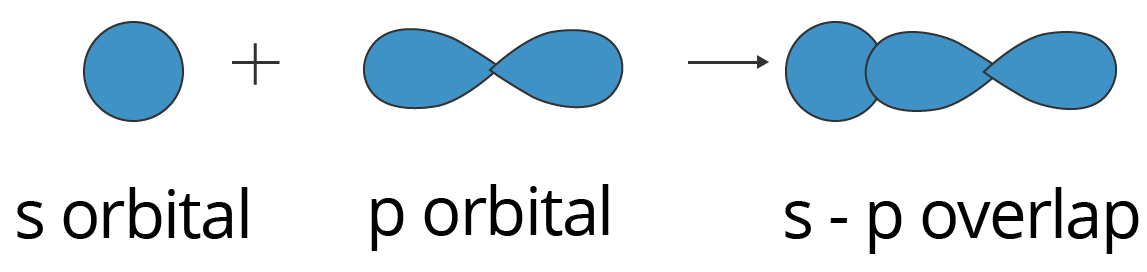Showing s-p Overlapping to form Sigma (σ) Bond

This type of overlapping can be observed in ammonia. An $\text{N}{{\text{H}}_{3}}$ molecule features 3 sigma bonds, formed by the overlap of the 2px, 2py, and 2pz orbitals belonging to the nitrogen atom and the 1s orbitals of the three hydrogen atoms.

P-P overlapping:

In this condition, one half-filled p orbital from each participating atom undergoes head-on overlapping along the internuclear axis. This type of overlapping is illustrated below.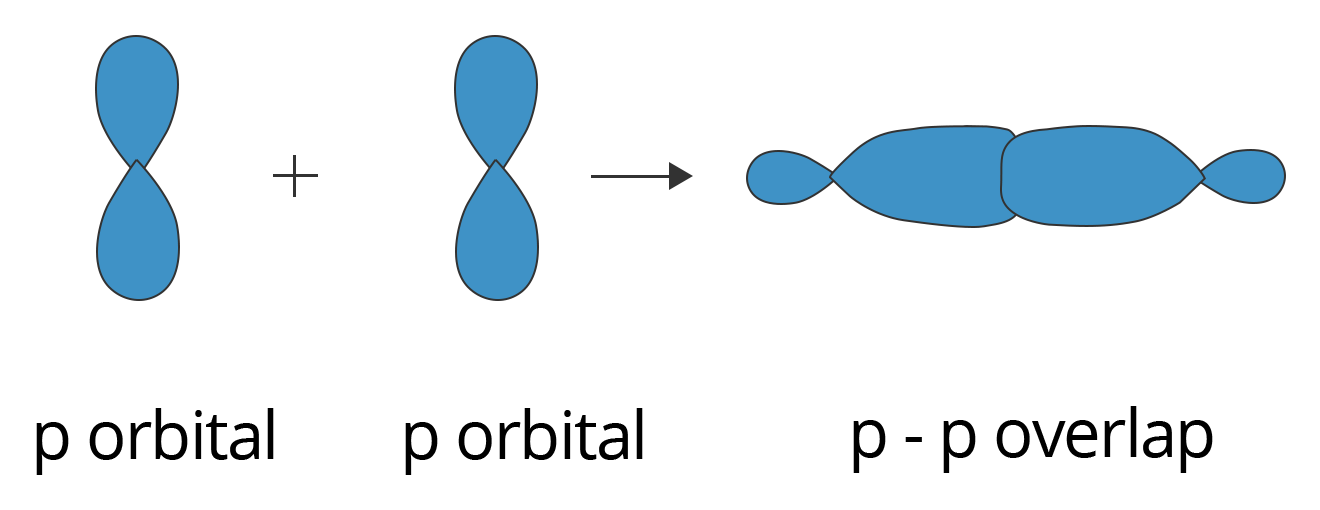Showing p-p Overlapping to form Sigma (σ) Bond

A $\text{C}{{\text{l}}_{2}}$ molecule features a p-p overlap of the 3pz orbitals of two chlorine atoms. It is important to note that the head-to-head overlapping of two p orbitals gives a sigma bond whereas the lateral overlap of these orbitals leads to the formation of pi bonds.

#### 2. The Pi (π) Bond:

Sidewise positive (same phase) overlap of atomic orbitals in a direction perpendicular to the internuclear axis forms pi bonds. The axes of the atomic orbitals are parallel to one another during bond formation, whereas the overlapping is perpendicular to the internuclear axis. This sort of covalent bonding is depicted in the diagram below.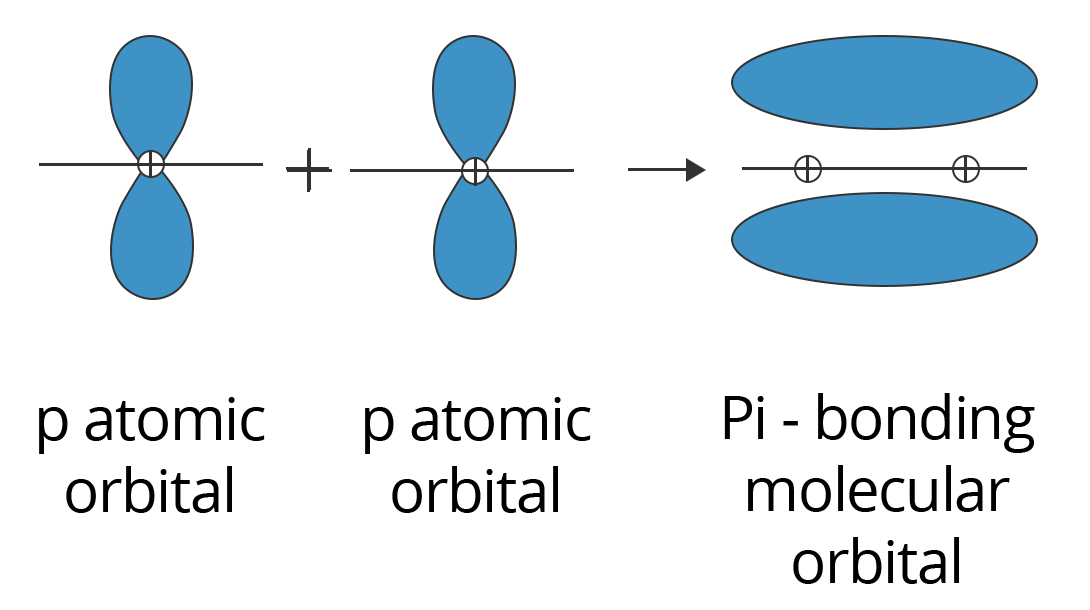Showing p-p Overlapping to form Pi (π) Bond

Due to the substantially smaller degree of overlapping, pi bonds are often weaker than sigma bonds. A typical triple bond is made up of two bonds and one sigma bond, whereas a typical double bond is made up of one sigma bond and one pi bond. It's worth noting that a sigma and pi bond combination is always stronger than a single sigma bond.

#### Difference Between Sigma and Pi Bonds:

The key Differences Between Sigma and Pi Bonds are Tabulated Below.

 Sigma Bond Pi Bond The overlapping orbitals can be pure or hybrid The overlapping orbitals must be unhybridized These bonds are strong and have high bond energies. These bonds are relatively weak. Can exist independently Must exist along with a sigma bond. Has an impact on the shape of molecules Has no role in determining the shape of molecules

#### Bond Parameters:

• Bond Length: The equilibrium distance between the centres of the nuclei of the two bonded atoms is called   its bond length.

• Bond Energy: The amount of energy required to break one mole of bonds of a particular type so as to separate them into gaseous atoms is called bond dissociation enthalpy or simply bond enthalpy.

• Bond Angle: The angle between the lines representing the directions of the bonds, i.e., the orbitals containing the bonding electrons is called the bond angle.

• Bond Order: In the Lewis representation of a molecule or ion, the number of bonds present between two atoms is called bond order.

### 11. Molecular Orbital Theory:

The orbitals of the connected atoms lose their distinct character and merge (overlap) to form bigger orbitals called molecular orbitals when the atoms to be joined get near together. A molecule has molecular orbitals, which are similar to atomic orbitals. The sole distinction is that electrons in atomic orbitals travel under the influence of only one nucleus (i.e. monocentric), whereas electrons in molecular orbitals move under the influence of numerous nuclei (polycentric).

#### Important Features of M.O.T:

1. Molecular orbitals exist around the nuclei of molecules, just as atomic orbitals exist around the nucleus of an atom.

2. The molecular orbitals are not the same as the atomic orbitals from which they are created.

3. In the molecular orbital, the constituent atoms' valence electrons are thought to be moving under the effect of the nuclei of participating atoms.

4. Like atomic orbitals in an isolated atom, molecule orbitals have varying energy levels.

5. The shape of molecular orbitals is determined by the forms of the atomic orbitals that they are created from.

6. Molecular orbitals, like atomic orbitals, are arranged in ascending energy order.

7. In bond formation, the number of molecular orbitals formed equals the number of atomic orbitals merging.

8. The Aufbau principle, Hund's rule, and Pauli's exclusion principle govern the filling of electrons in molecular orbitals, just as they do in atomic orbitals.

#### Conditions for Atomic Orbitals to Form M.O:

• The combining A.O. should be of comparable energy.

• The combining atomic orbitals must overlap to a large extent greater the overlap, stable is the molecule formed.

### 12. Metallic Bond:

Metal atoms are the constituent particles of metallic solids, which are bound together by metallic bonds. A metal atom is said to be made up of two parts: valence electrons and the remaining kernel (the nucleus and inner shells). Metal atom kernels occupy the lattice sites, while valence electrons occupy the space between the kernels. Metal atoms' valence electrons are not retained firmly by the nucleus due to low ionisation energy. As a result, the electrons leave one kernel's influence field and enter the influence field of another. As a result, the electrons are movable rather than localised. The simultaneous attraction between the kernels and the mobile electrons which hold the kernel together is known as metallic bond. This model is known as the electron sea model.

### 13. Hydrogen Bonding:

Hydrogen bonding refers to the formation of Hydrogen bonds, which are a special class of attractive intermolecular forces that arise due to the dipole-dipole interaction between a hydrogen atom that is bonded to a highly electronegative atom and another highly electronegative atom which lies in the vicinity of the hydrogen atom. For example, in water molecules $\left( {{\text{H}}_{\text{2}}}\text{O} \right)$, hydrogen is covalently bonded to the more electronegative oxygen atom. Therefore, hydrogen bonding arises in water molecules due to the dipole-dipole interactions between the hydrogen atom of one water molecule and the oxygen atom of another $H_2O$ molecule.

Here, the location of the bond pair of electrons in the O-H bond is very close to the oxygen nucleus (due to the large difference in the electronegativities of oxygen and hydrogen). Therefore, the oxygen atom develops a partial negative charge (-δ) and the hydrogen atom develops a partial positive charge (+δ). Now, hydrogen bonding can occur due to the electrostatic attraction between the hydrogen atom of one water molecule (with +δ charge) and the oxygen atom of another water molecule (with -δ charge). Thus, hydrogen bonds are a very special class of intermolecular attractive forces that arise only in compounds featuring hydrogen atoms bonded to a highly electronegative atom. Hydrogen bonds are mostly strong in comparison to normal dipole-dipole and dispersion forces. However, they are weak compared to true covalent or ionic bonds.

#### Conditions for Hydrogen Bonding:

The Conditions for Hydrogen Bonding are:

• The molecule must contain a highly electronegative atom linked to the hydrogen atom. The higher the electronegativity, the more is the polarization of the molecule.

• The size of the electronegative atom should be small. The smaller the size, the greater the electrostatic attraction.

#### Types of Hydrogen Bonding:

There are two types of H bonds, and it is classified as the following:

• Intermolecular Hydrogen Bonding

When hydrogen bonding takes place between different molecules of the same or different compounds, it is called intermolecular hydrogen bonding.

For example – hydrogen bonding in water, alcohol, ammonia etc.

• Intramolecular Hydrogen Bonding:

The hydrogen bonding which takes place within a molecule itself is called intramolecular hydrogen bonding.

It takes place in compounds containing two groups such that one group contains hydrogen atom linked to an electronegative atom and the other group contains a highly electronegative atom linked to a lesser electronegative atom of the other group.

The bond is formed between the hydrogen atoms of one group with the more electronegative atom of the other group.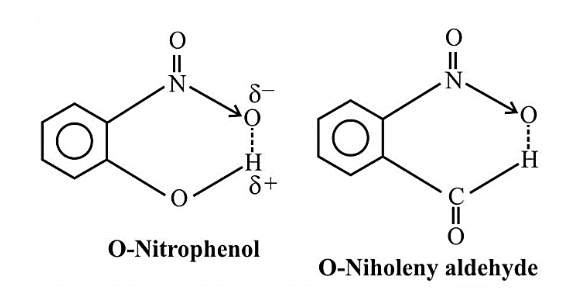Showing Intramolecular Hydrogen Bonding

#### 14. Van Der Waals Forces:

Van der Waals forces are weak intermolecular forces that are dependent on the distance between atoms or molecules. These forces arise from the interactions between uncharged atoms/molecules.

For example, Van der Waals forces can arise from the fluctuation in the polarizations of two particles that are close to each other.

In the group of forces that fall under the category of ‘weak chemical forces’, Van der Waals forces are the weakest. They are known to rapidly vanish when the distance between the interacting molecules increases. The strengths of Van der Waals forces typically range from 0.4 kJ.mol-1 to 4 kJ.mol-1.

When the electron density around the nucleus of an atom undergoes a transient shift, it is common for Van der Waals forces to arise. For example, when the electron density increases in one side of the nucleus, the resulting transient charge may attract or repel a neighbouring atom. The nature of these forces is dependent on the distance between the atoms:

• When the distance between the atoms is greater than 0.6 nanometres, the forces are extremely weak and cannot be observed.

• When the distance between the atoms ranges from 0.6 to 0.4 nanometres, the forces are attractive.

• If the interatomic distance is smaller than 0.4 nanometres, the forces are repulsive in nature.

Vander Waals forces are weak intermolecular forces that are dependent on the distance between atoms or molecules. These forces arise from the interactions between uncharged atoms/molecules.

For example, Van der Waals forces can arise from the fluctuation in the polarizations of two particles that are close to each other.

In the group of forces that fall under the category of ‘weak chemical forces’, Van der Waals forces are the weakest. They are known to rapidly vanish when the distance between the interacting molecules increases. The strengths of Van der Waals forces typically range from 0.4 kJ.mol-1 to 4 kJ.mol-1.

When the electron density around the nucleus of an atom undergoes a transient shift, it is common for Van der Waals forces to arise. For example, when the electron density increases in one side of the nucleus, the resulting transient charge may attract or repel a neighbouring atom. The nature of these forces is dependent on the distance between the atoms:

• When the distance between the atoms is greater than 0.6 nanometres, the forces are extremely weak and cannot be observed.

When the distance between the atoms ranges from 0.6 to 0.4 nanometres, the forces are attractive.

• If the interatomic distance is smaller than 0.4 nanometres, the forces are repulsive in nature.

#### Types of Vander Waal’s Force:

1. Ion Dipole Attraction - This force is between an ion such as $\text{N}{{\text{a}}^{+}}$ and a polar molecule such as HCl.

2. Dipole Dipole Attraction - It is again in between two polar molecules such as HF and HCl.

3. Ion Induced Dipole Attraction - In this case a neutral molecule is induced by an ion as a dipol.

4. Dipole - Induced dipole attraction: In this case a neutral molecule is induced as a dipole by another dipole.

5. Induced Dipole - Induced dipole attraction or London dispersion force between two nonpolar molecules as in $\text{C}{{\text{l}}_{2}}$, He etc.

### 15. Dipole Moment:

Dipole moments in chemistry express the polar character or polarity of the molecules. The product of charge and distance of separation of atoms in a chemical bond defines the term dipole moment. If +q amount positive charge separated by -q amount of negative charge by the bond distance I, then dipole moment $(\mu)$ of polar molecule $=\mathrm{q} \times \mathrm{I} .$ Perfectly non-polar molecules like hydrogen, oxygen, and nitrogen have zero dipole moment and the polar molecules like water, ammonia, and methane have positive values. It is a tool used to calculate the percentage ionic character, electric polarization, and residual charge on the atoms of the molecules.

#### Unit of Dipole Moment:

$\mu=\mathrm{q} \times \mathrm{d}$

The charge q on an electron is $4.8\times 10^{-10}$ esu. The distance is measured in $\mathrm{cm}$ and is of order of 1 Å, i.e., $10^{-8} \mathrm{~cm}$. Its unit in CGS system is debye (D).

A molecule will have a dipole moment of 1 Debye (D) if charges of $1 \times 10^{-8}$ esu are separated by a distance of 1Å Thus, $1 \mathrm{D}=1 \times 10^{-18}$ esu. $\mathrm{cm}$.

#### Applications of Dipole Moment:

1. For predicting the nature of the molecules- molecules with zero dipole moment are non- polar while the molecule with specific dipole moment are polar in nature.

2. For determining the shape of the molecule-If a molecule has a specific dipole moment then its shape will not be symmetrical, they may be bent or angular and the molecule with zero dipole moment will be symmetrical and has linear shape.

3. For comparing the polarities of the molecules- greater the dipole moment value, more will be the polarity and vice versa.

### Solved Example:

1. Which of the following compounds has the largest dipole moment?

(i) $\mathbf{C H}_{3} \mathrm{OH}$

(ii) $\mathbf{C H}_{4}$

(iii) $\mathbf{C F}_{4}$

(iv) $\mathrm{CO}_{2}$

(v) $\mathbf{C H}_{3} \mathbf{F}$.

Ans: $\mathrm{CH}_{4}$ and $\mathrm{CF}_{4}$ have tetrahedral structure and are symmetrical, hence their dipole moment is zero, $\mathrm{CO}_{2}$ is linear and hence its dipole moment is also zero of the remaining $\mathrm{CH}_{3} \mathrm{OH}$ and $\mathrm{CH}_{3} \mathrm{~F}$, since $\mathrm{F}$ is more electronegative than $\mathrm{O}, \mathrm{CH}_{3} \mathrm{~F}$ will have high dipole moment.

2. What type of bond is formed when atoms have

(i) Zero difference of electronegativity.

(ii) Little difference in electronegativity.

(iii) High difference of electronegativity.

Sol. (i) Non-polar covalent

(ii) Polar covalent

(iii) Electro-valent.

3. Explain the formation of a chemical bond.

Sol. Mutual sharing or the transfer of one or more electrons create a chemical bond. Each combining atom develops a stable noble gas electronic structure with 8 electrons in its outermost shell as a result of this process.

4. Iodine $\left(\mathrm{I}_{2}\right)$ is a volatile solid at room temperature and has very low solubility in water. However, $\mathrm{I}_{2}$ is highly soluble in an aqueous solution of KI. Explain.

Sol. $\mathrm{I}_{2}(\mathrm{~s})$ being a molecular solids, the molecules are completely nonpolar and covalent, and have very low affinity for polar solvents like water. However, in solution of KI, I $_{2}$ forms forms $\mathrm{KI}_{3}$ salt as

$\mathrm{KI}+\mathrm{I}_{2} \longrightarrow \mathrm{KI}_{3}$

$\mathrm{KI}_{3}$, a strongly ionic salt dissolves easily in water like other normal salts of the alkali metals. Hence, $\mathrm{I}_{2}$ becomes soluble in $\mathrm{KI}$ via $\mathrm{KI}_{3}$.

## FAQs on Chemical Bonding and Molecular Structure Class 11 Notes CBSE Chemistry Chapter 4 (Free PDF Download)

1. Which are some crucial questions that come in the exam from Class 11 Chapter 4?

Some important questions of Chapter 4 Chemistry Class 11.

• Define a chemical bond.

• What is the main feature of the Lewis approach of chemical bonding?

• Define the octet rule.

• Define an ionic bonding.

• Define bond order.

• What type of bond is formed when the atoms have a high difference of electronegativity?

• Define the dipole moment.

• Write the resonating structure of the O3 molecule.

• Define hybridisation

By solving these questions, the student can become more confident and can perform in a better way during the main exam.

2. Why are Class 11 Chapter 4 revision notes essential for students?

Keeping revision notes of Chapter 4 Class 11 Chemistry becomes essential during the exam because it's nearly impossible to read everything from inside the books. Hence, it would help if you had the revision notes where all the vital things jotted down together for better understanding and the convenience of the student. The revision notes also work as a practice paper for the students.

3. What is chemical bonding in Class 11?

The bonding in which different atoms combine with the help of a force to form ions, crystals, molecules or other stable chemical compounds is known as chemical bonding. For example, carbon dioxide, lithium fluoride, water, sodium chloride, etc. All these chemical compounds are formed due to chemical bonding. The forces included in this bonding are weak and strong intramolecular forces. As the bond formation takes place by sharing of the electrons, the different types of chemical bonds are:

• Covalent Bond

• Ionic Bond

• Polar Bond.

4. What are the topics in chemical bonding Class 11?

The different topics covered in Chapter 4 of Class 11 Chemistry are:

• Limitations of Octet Rule

• Types of Bonds

• Bonds Parameters

• How to draw and write a Lewis Structure

• Formal Charge Formula

• Lattice Enthalpy

• Resonance and Resonating Structures

• Bonds Polarity

• Overlapping of Orbitals

• VSEPR Theory

• Hybridisation and its Types

• Valence Band Theory

• Molecular Orbital Theory

• Bonds of Hydrogen

• Types of Hydrogen Bonding

All the above-mentioned topics are explained in detail in the NCERT book. To get the notes on these topics free of cost, refer to the page Revision Notes For NCERT Class 11 Chemistry.

5. Which chapter is chemical bonding?

Chemical Bonding is Chapter 4 of Class 11 Chemistry. The main idea behind this chapter is the formation of chemical bonds and the molecular structure.  This chapter also tells us about the molecules or atoms and their various structures through examples and diagrams. Also, different types of bonds are explained in this chapter. Solving the NCERT questions will help students to comprehend the concepts more easily.  The notes of this chapter are available in the form of PDF files on the website of Vedantu (vedantu.com).

6. What is bond angle in chemistry class 11?

The bond angle is one of the bond parameters mentioned in Chapter 4 of Class 11 Chemistry. It is defined as the average angle measured between the orbitals of the central atom. The bond angle is measured in degrees. For example, the bond angle in carbon dioxide is 180 degrees because of its linear shape. As the orbitals contain the electron pairs, the repulsion or attraction between the bonded electron pairs or lone pairs decides the bond angle of the chemical compound. To know more download the Vedantu app.

7. What do you mean by hydrogen bonding? Also, explain its types.

The bonding done by the electrostatic force of attraction between the hydrogen atom and the most electronegative atom like fluorine, oxygen and nitrogen is known as hydrogen bonding. Examples of hydrogen bonds are ammonia, hydrofluoric acid, etc. There are two types of hydrogen bonds:

• Intramolecular Hydrogen Bonding - This bond formation takes place within the molecule itself.

• Intermolecular Hydrogen Bonding - This bond is present between the different molecules of different or same compounds.

Among these bonds, the intramolecular bond is the strongest.